# Kernel convolution, for image processing

Those filters we see over pictures on some popular apps, many are base on a method called Kernel Convolution, effects like Gaussian blur, image Embossing etc. can be achieve using this. The method consist on applying a operation on every pixel of the image based on a kernel, a matrix with values.

the kernel or convolution matrix is normally small, software like Gimp uses 3x3 and 5x5 kernels, an the process can be seen as placing the center of the convolution matrix in every pixel, and calculating the sum of the multiplication of every pixel of the convolution matrix times the value under the corresponding cell in the second matrix(or image)that final value is then divided by either the sum of the values of the convolution matrix or a specific number: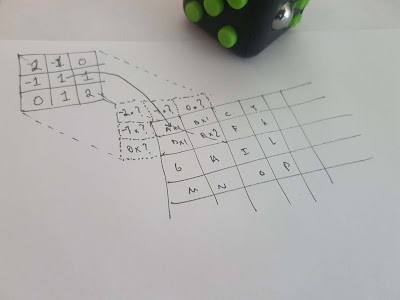There are some special cases on the borders of the second matrix,(or image)since the cell on the kernel matrix doesn't match with any cell on the second matrix, but of course, there are some methods to avoid the problem, Extend (the one used in this software), wrap, mirror or crop. For the software the approach is simple, the convolution matrix is a matrix of floats, and the second matrix is represented as a matrix of integer values, this second matrix will be created based on the values of a specific color in an image, either red, green or blue, so that the filter can be applied to a specific channel. This matrix then will be used to replace the values on the image for the color it represents. The convolution function looks like this:

``````
public static int[][] convolution(float [][] convolutionMatrix, int[][] matrix, int sum){
int widthConvolution = convolutionMatrix.length;
int heightConvolution = convolutionMatrix.length;
int widthMatrix = matrix.length;
int heightMatrix = matrix.length;
if(!validateConvolutionMatrixDimension(widthConvolution,heightConvolution))return null;
int convolutionMatrixSum = (sum == 0)?128:sum; //normalize
if(convolutionMatrixSum < 0)convolutionMatrixSum = 255+convolutionMatrixSum;
int convolutionDistanceFromCenter = widthConvolution / 2;
int resultMatrix[][] = new int[heightMatrix][widthMatrix];
for(int heightCounter = 0; heightCounter < heightMatrix; heightCounter++){
for(int widthCounter = 0; widthCounter < widthMatrix; widthCounter++){
long preValue = 0;
for(int i = 0; i < heightConvolution; i++){
for(int j = 0; j < widthConvolution; j++){
int rowInMatrix = heightCounter - (convolutionDistanceFromCenter - i);
int columnInMatrix= widthCounter - (convolutionDistanceFromCenter - j);
if(columnInMatrix < 0){
columnInMatrix = 0;
}else if(columnInMatrix >= widthMatrix)columnInMatrix = widthMatrix -1;
if(rowInMatrix < 0){
rowInMatrix = 0;
}else if(rowInMatrix >= heightMatrix)rowInMatrix = heightMatrix-1;
preValue += convolutionMatrix[i][j]*matrix[rowInMatrix][columnInMatrix];
int colorValue = (int)(preValue / convolutionMatrixSum);
resultMatrix[heightCounter][widthCounter] = (colorValue < 0)?0:(colorValue >255)?255:colorValue;
}
}
}
}
return resultMatrix;
}
``````

Some normalization is applied, it consist in avoid a division by zero by applying an offset of 128 (like Gimp does), and if it is negative an offset of 255 to avoid negative invalid pixel values, also if the pixel value after the transformation is less than zero its converted to zero, and if it is greater than 255 is converted to 255, (minimum and maximum value for a pixel color). Some images are needed to test the program and this cc0 images serve the purpose:

Original: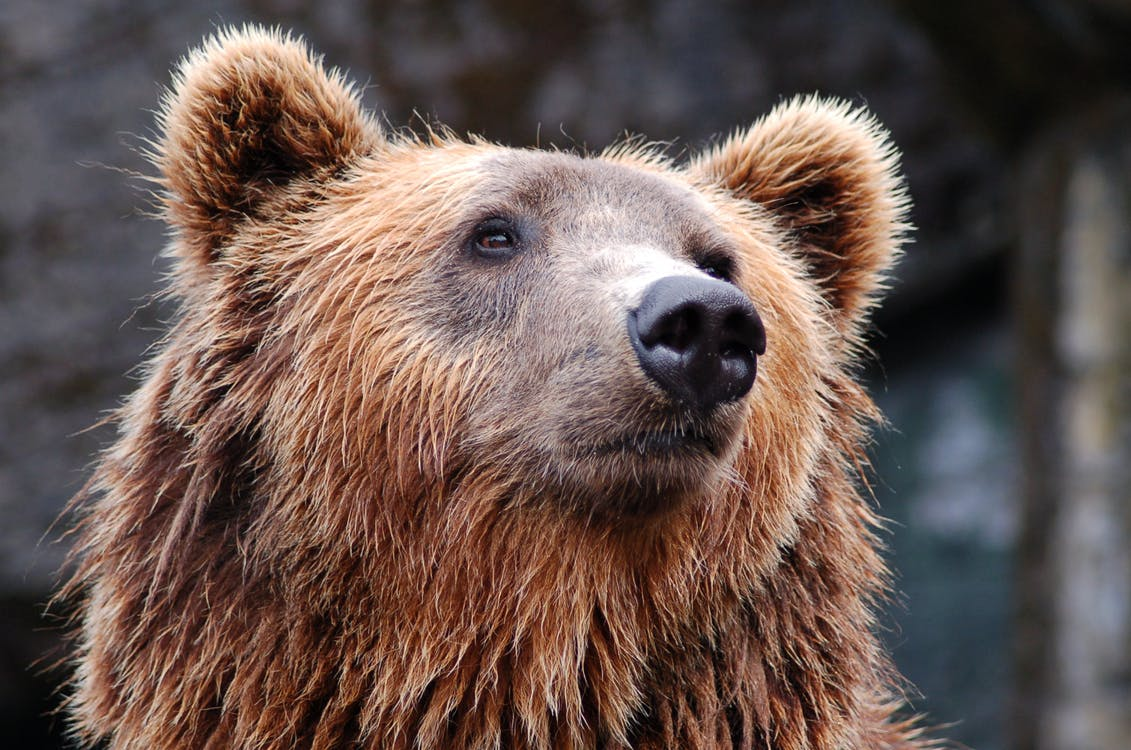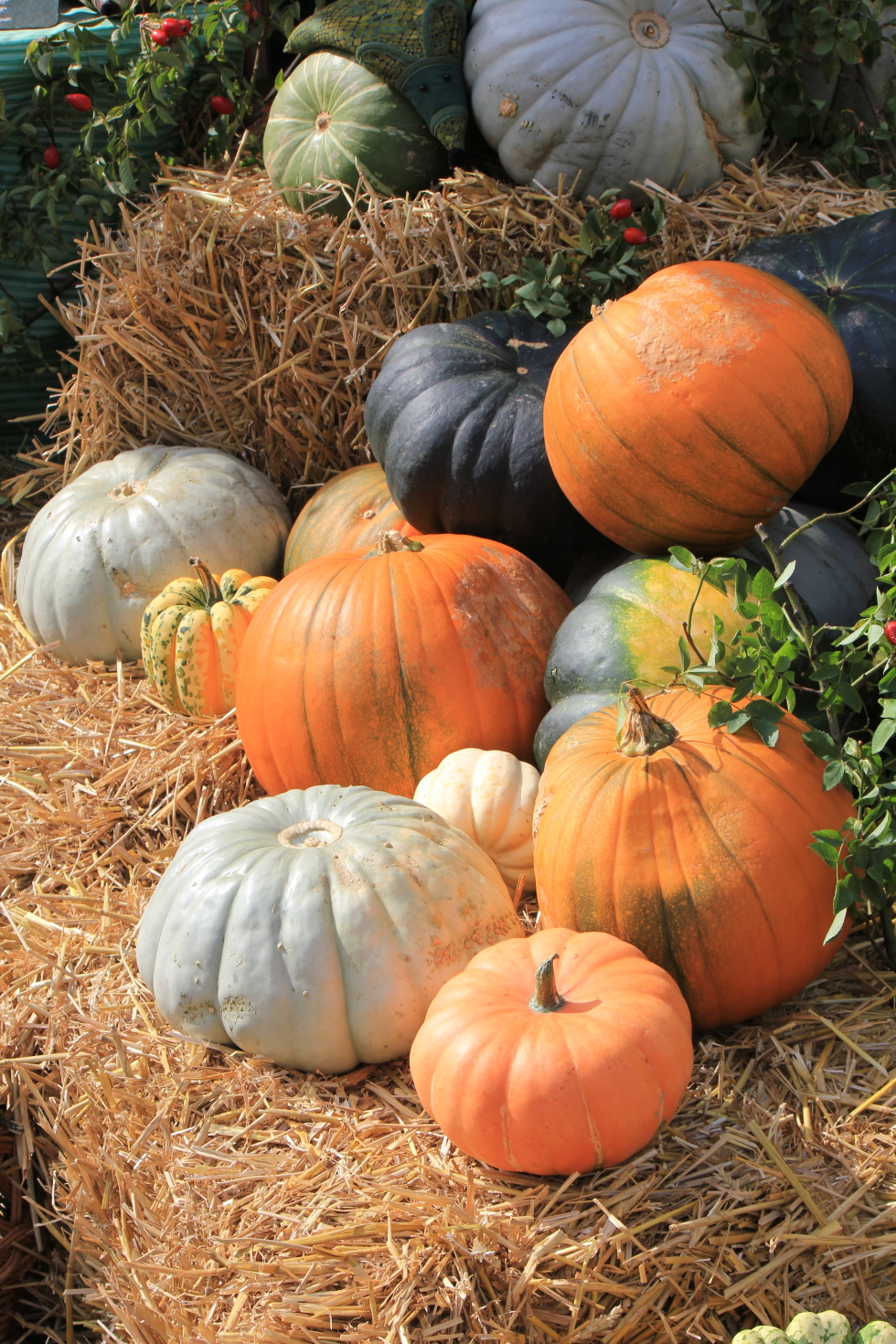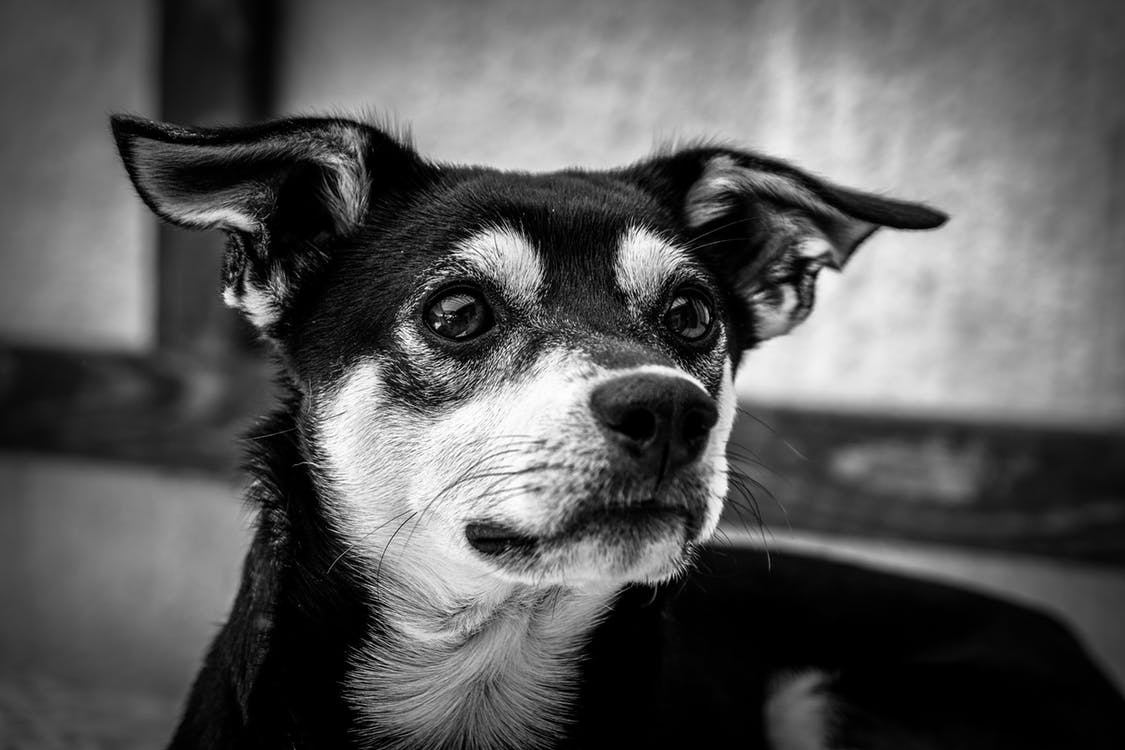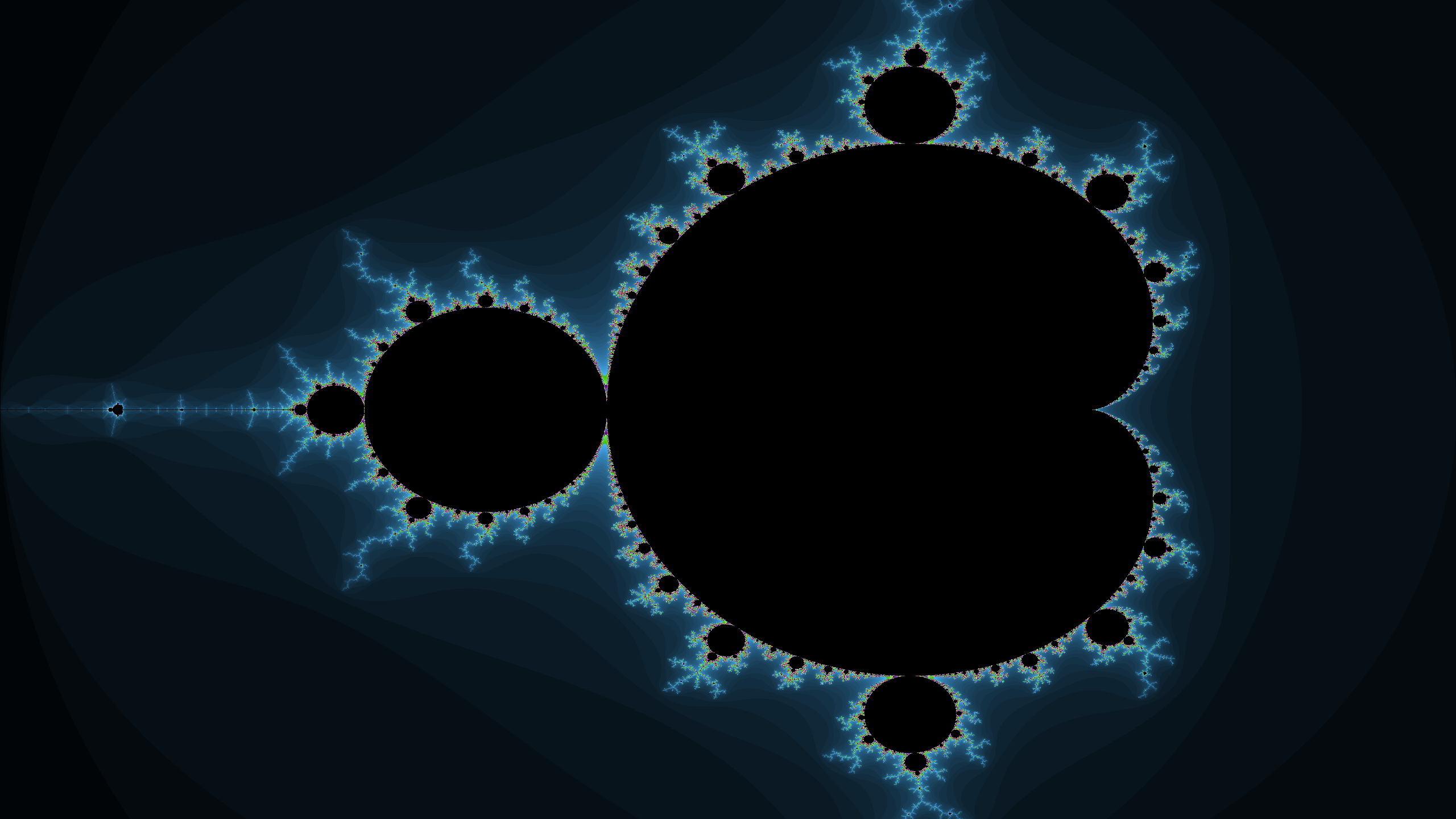Edge Detect:

``````
public static float[][] edgeDetectGimp = {
{0,1,0},
{1,-4,1},
{0,1,0}
};
``````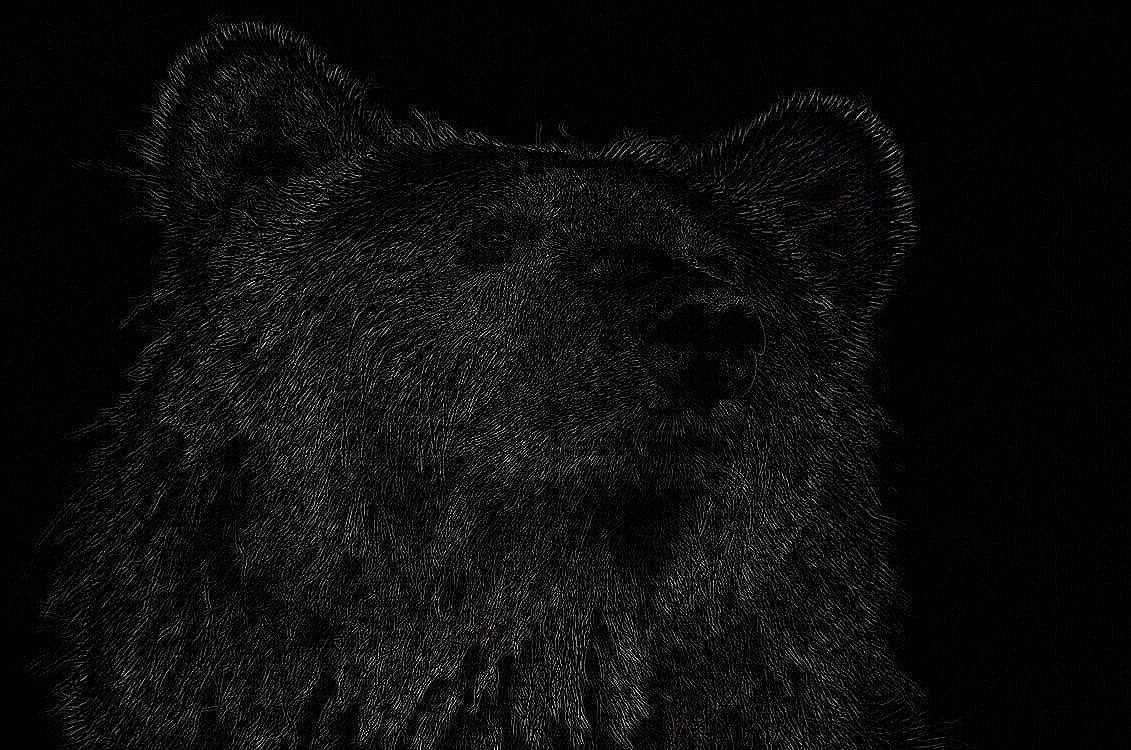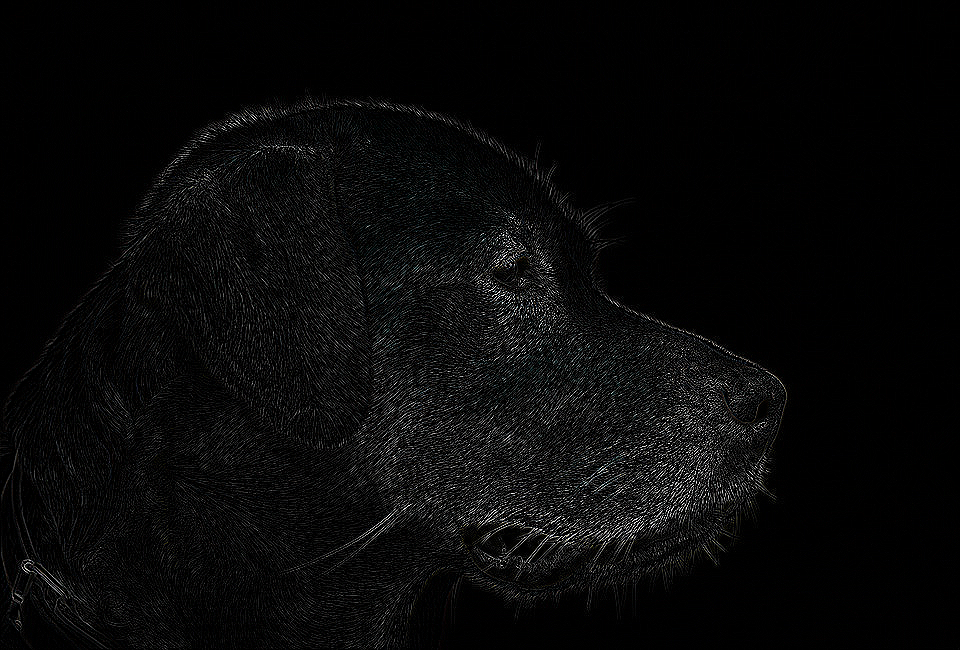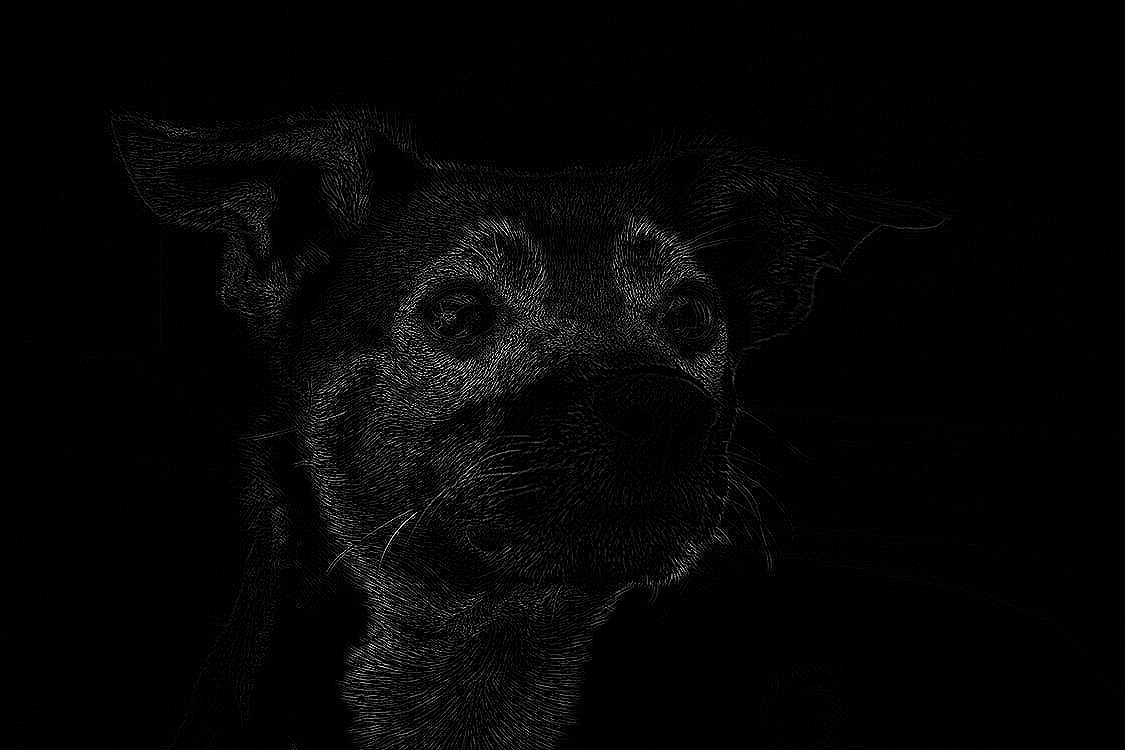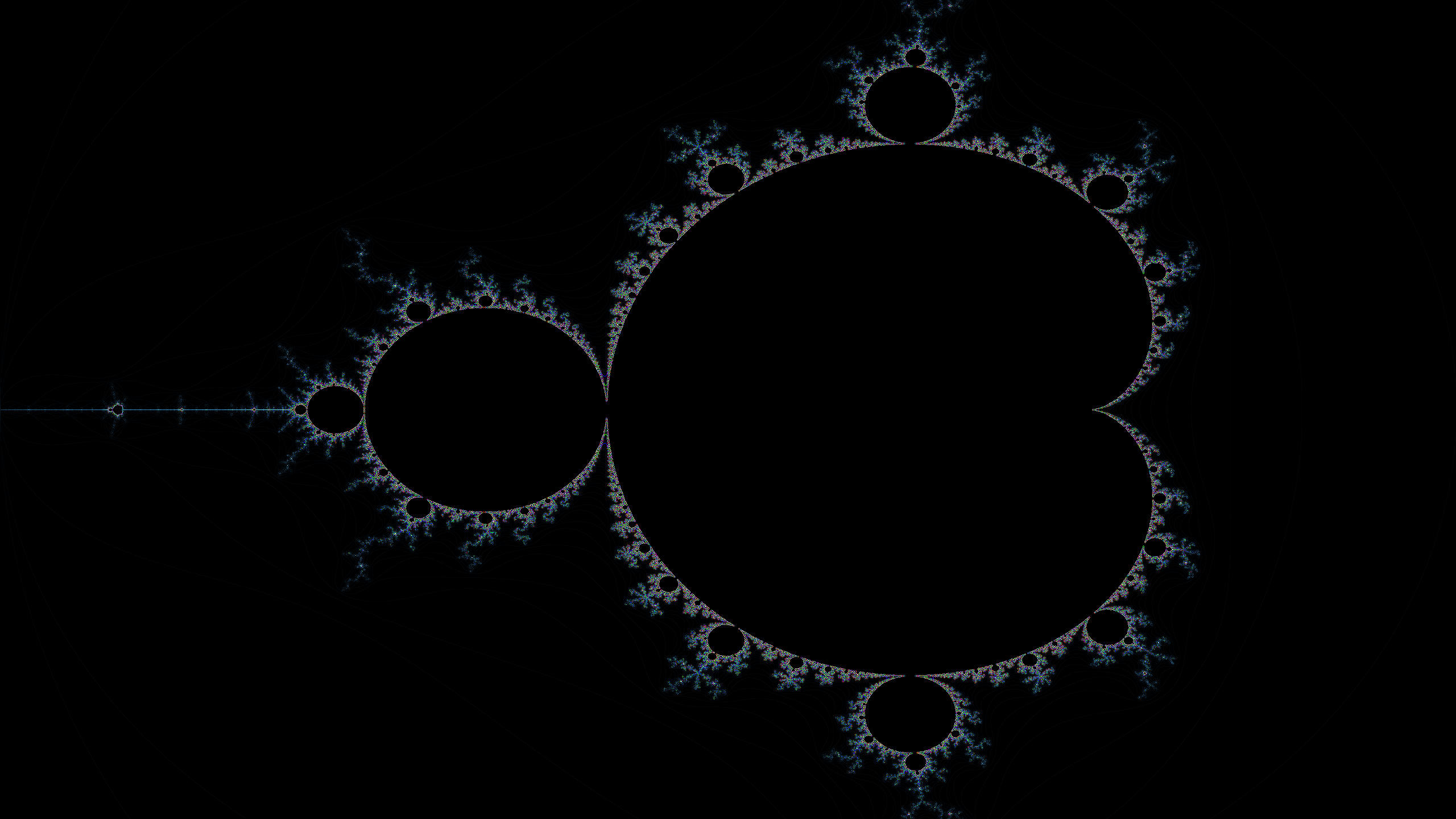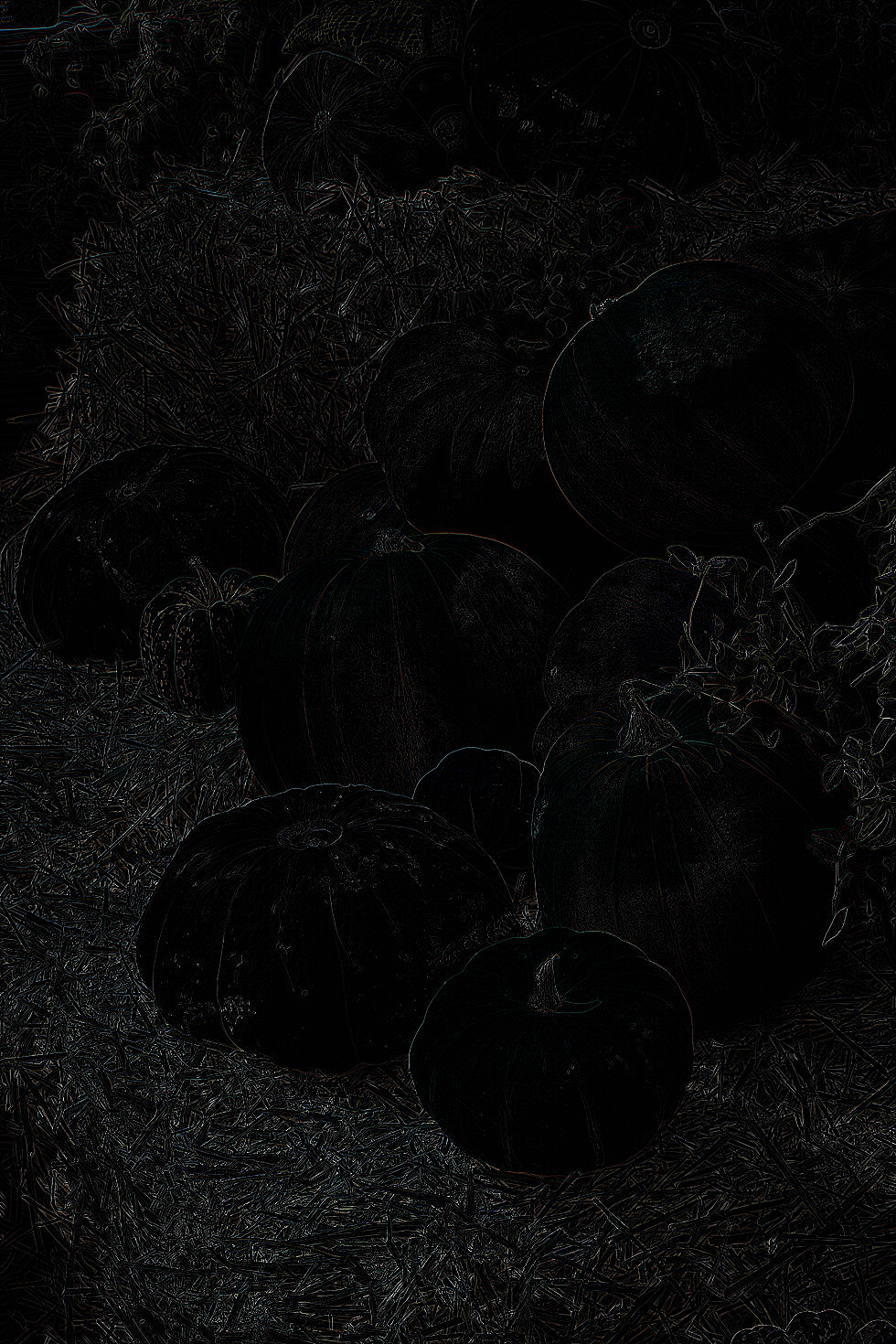Emboss:

``````
public static float[][] emboss = {
{-2,-1,0},
{-1,1,1},
{0,1,2}
};
``````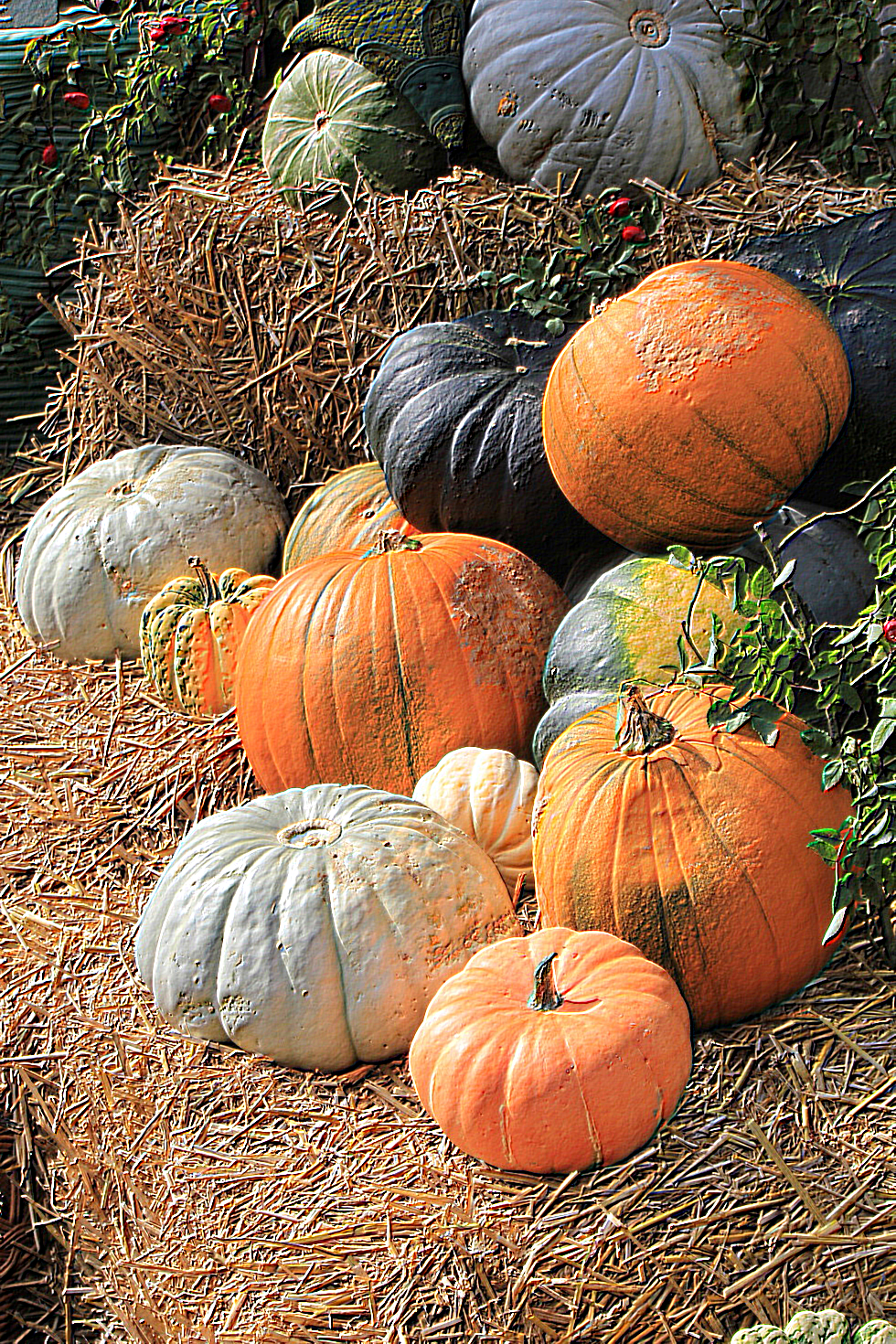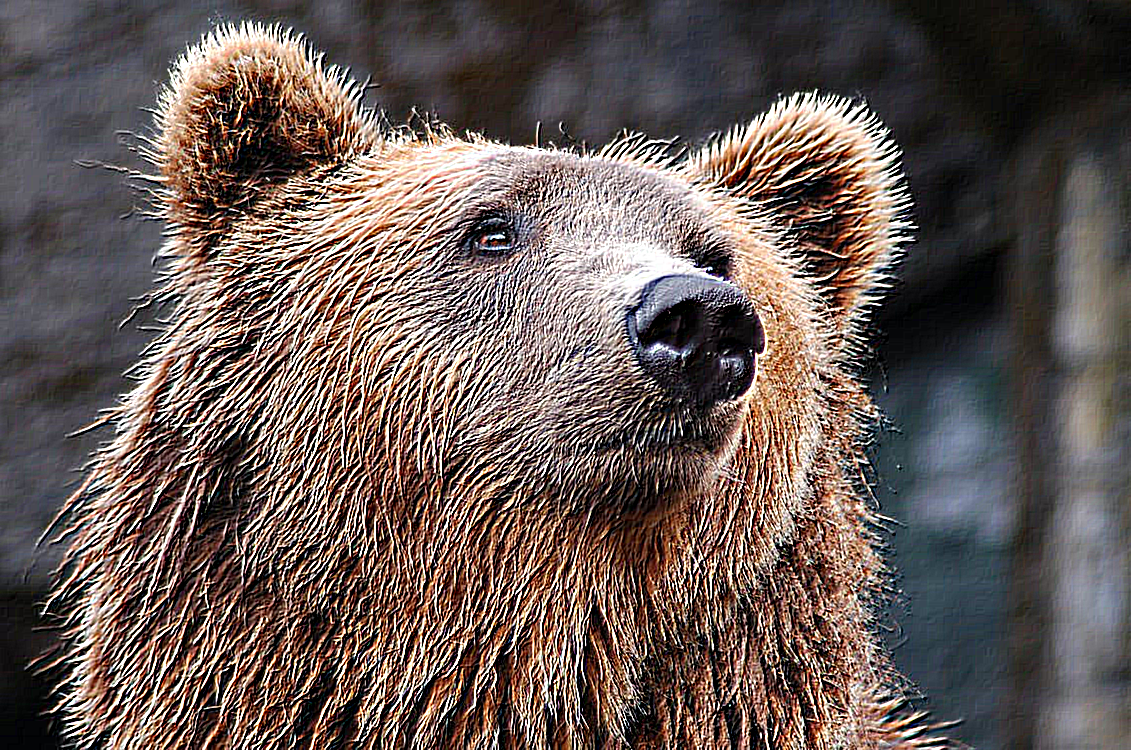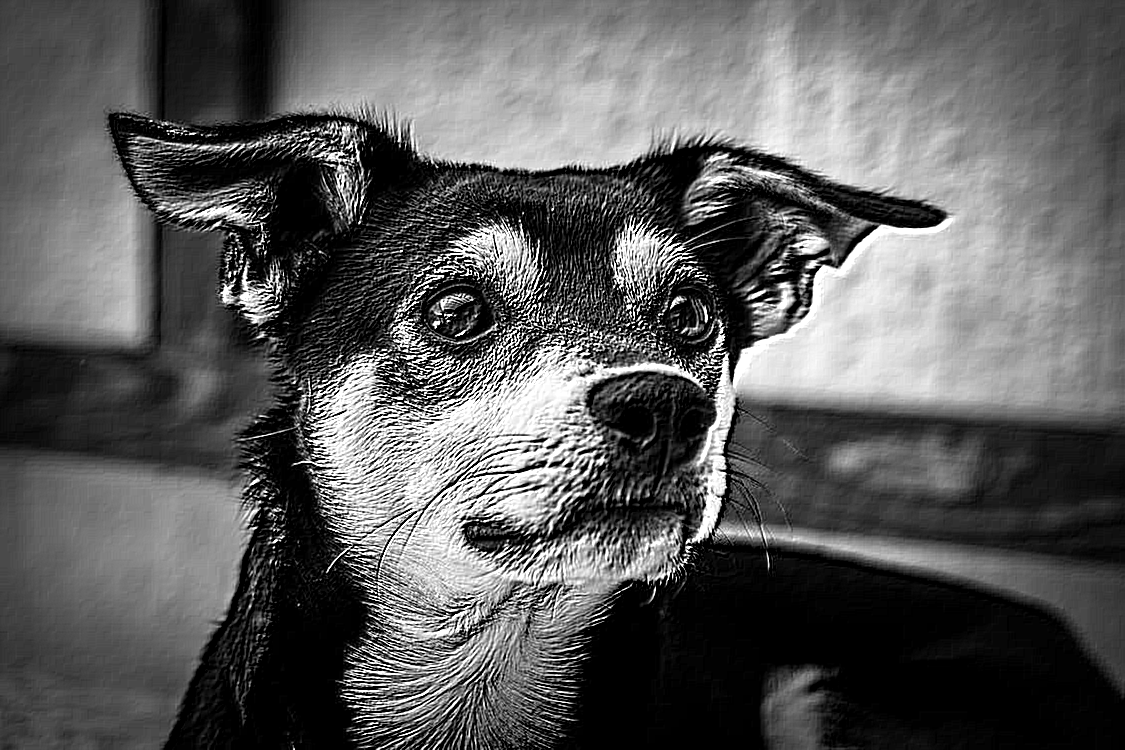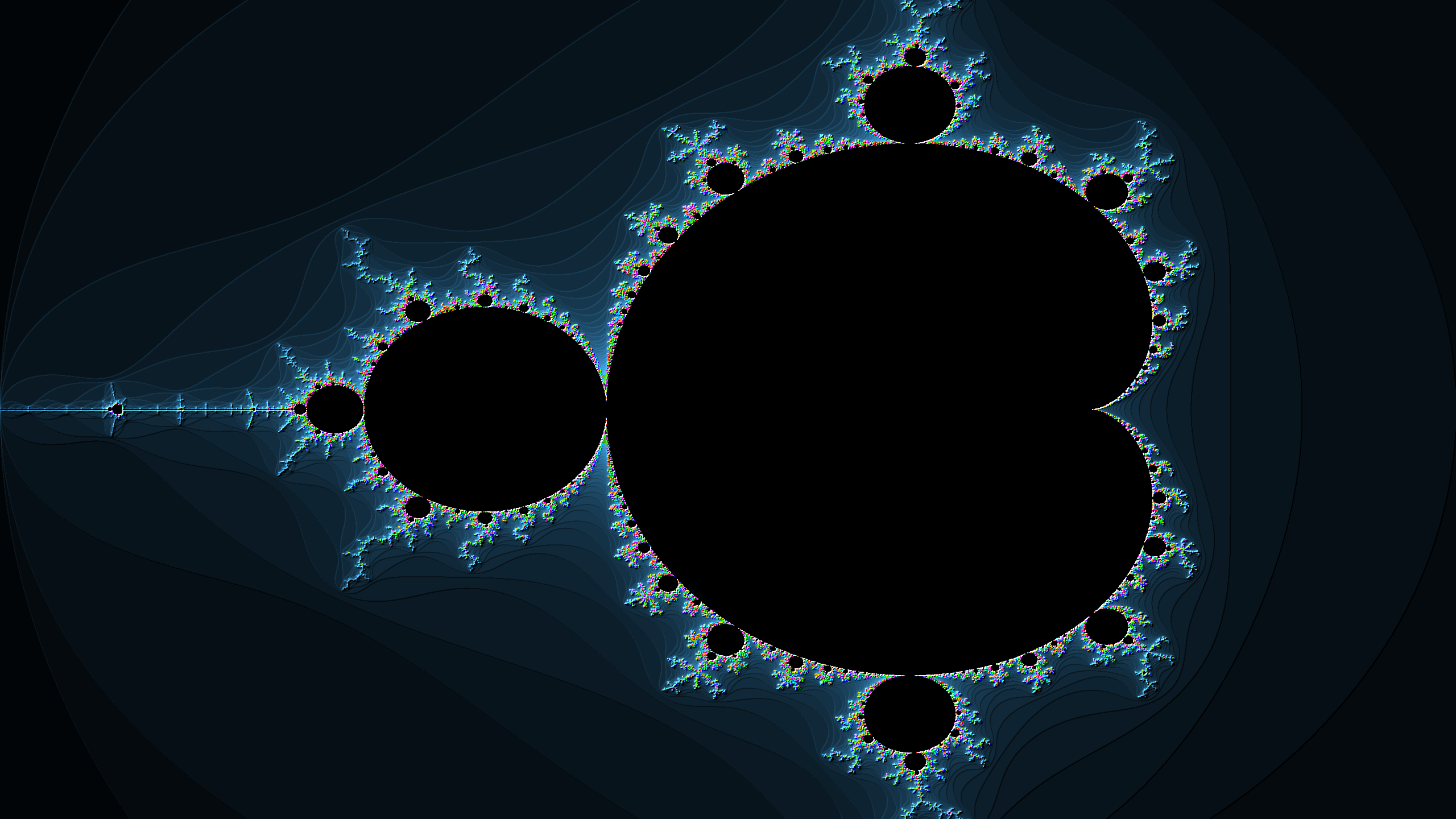Edge Enhancement:

``````
public static float[][] edgeEnhanceGimp = {
{0,0,0},
{-1,1,0},
{0,0,0}
};
``````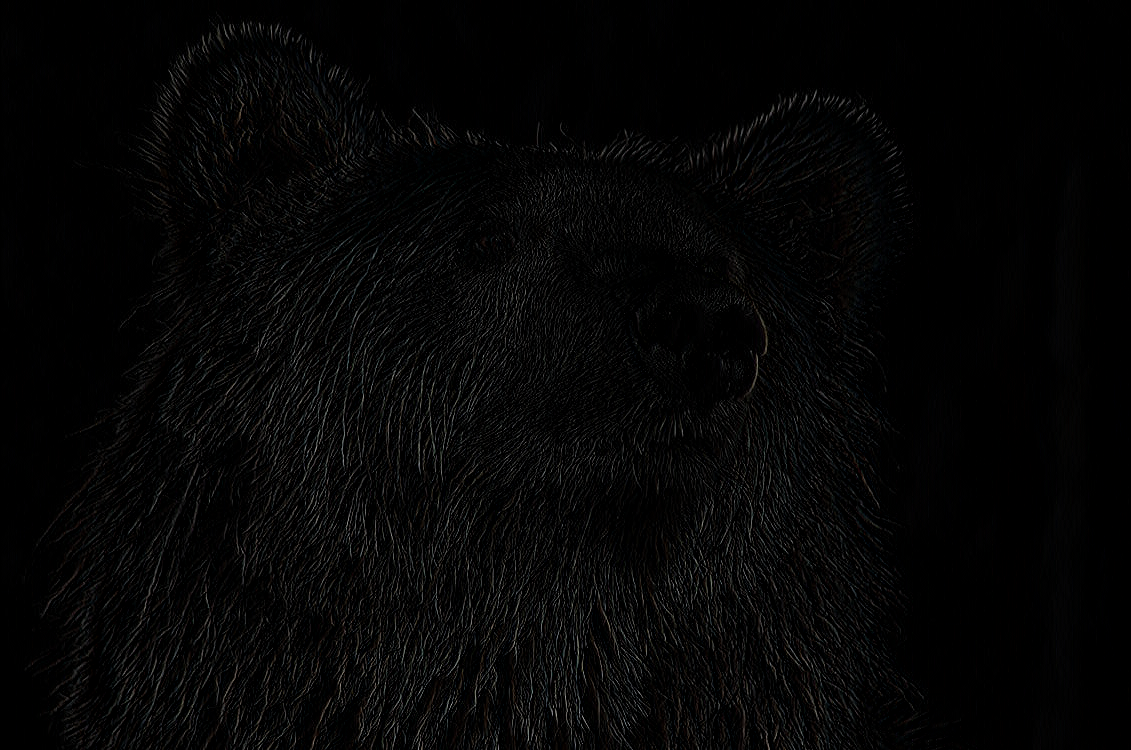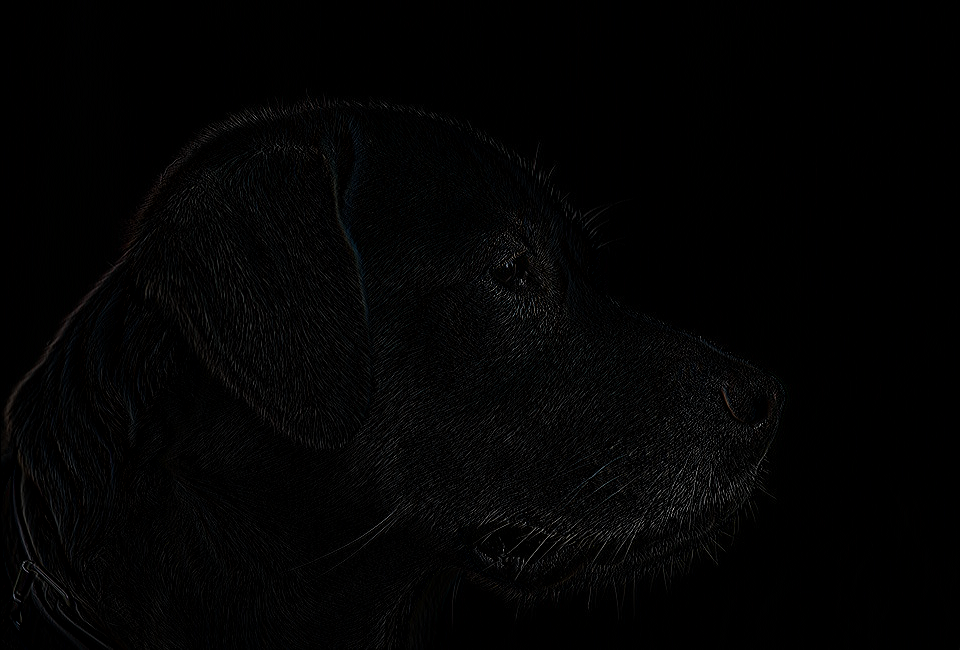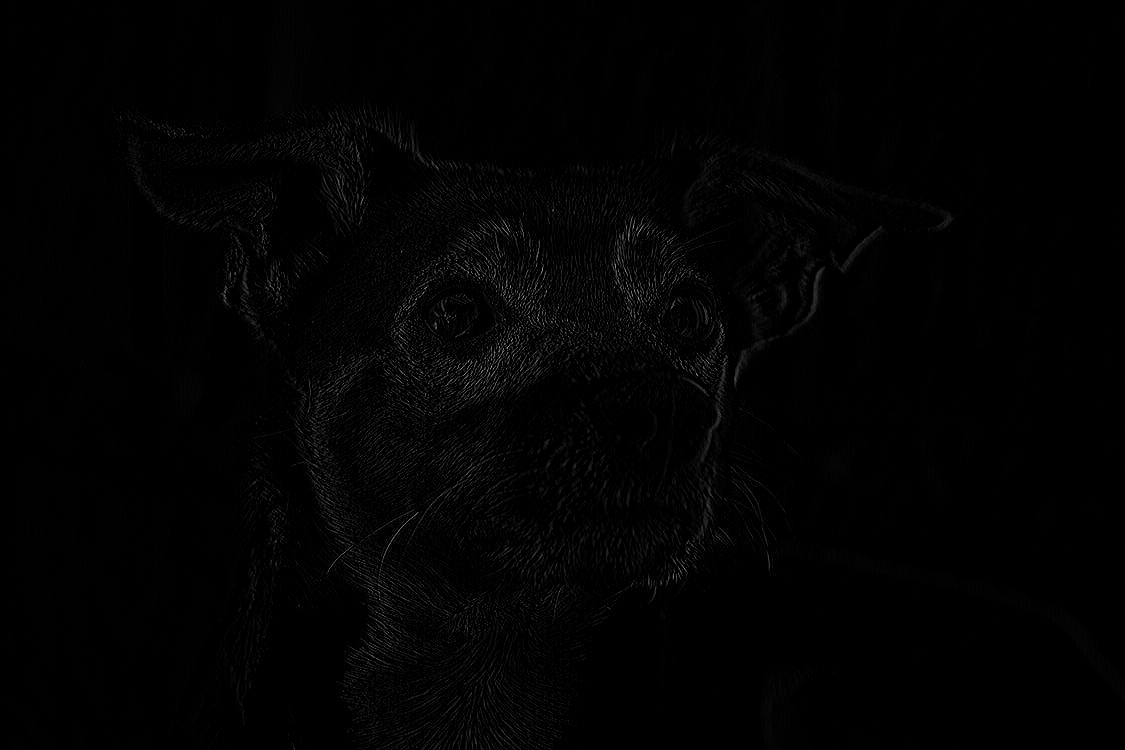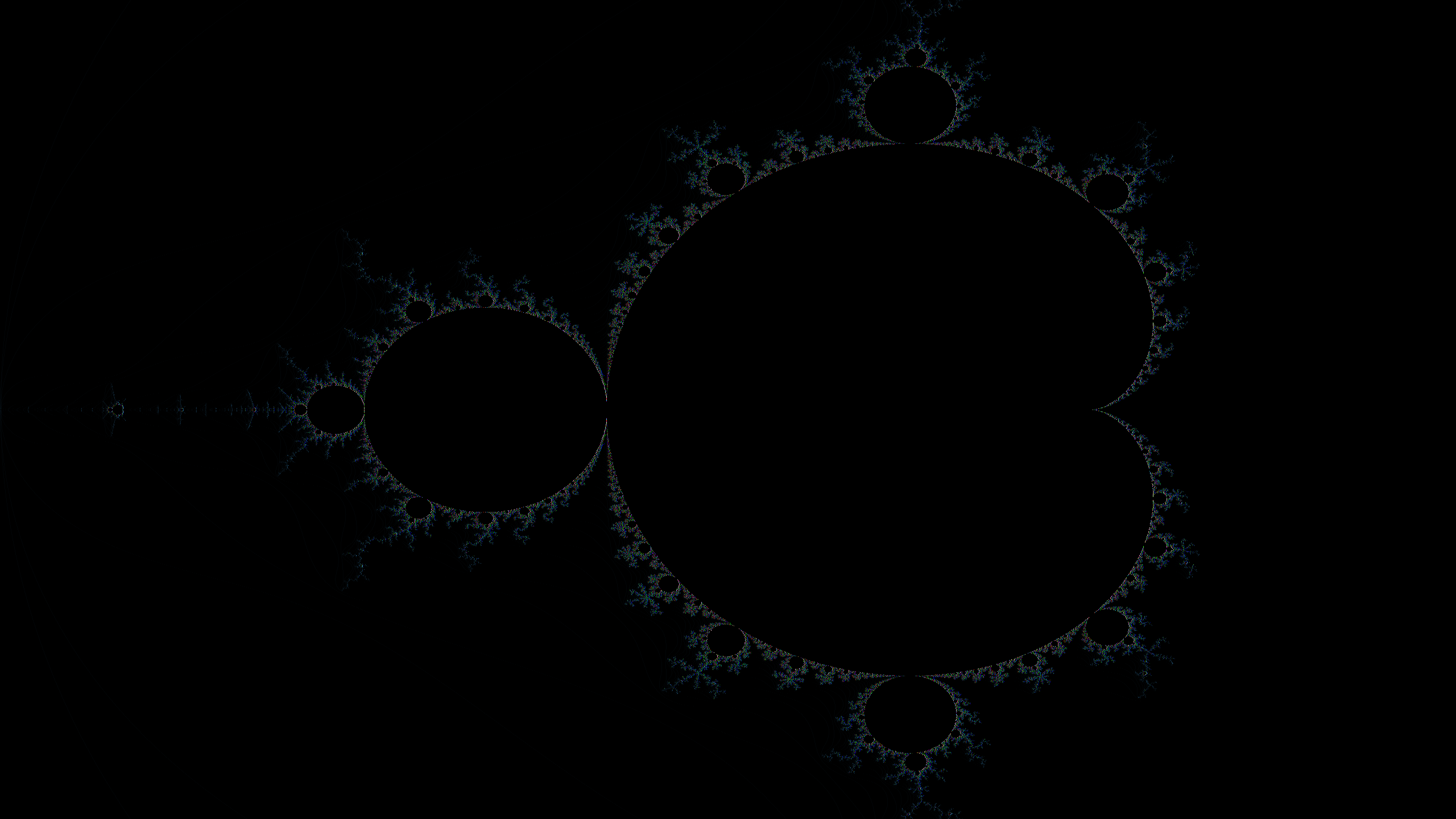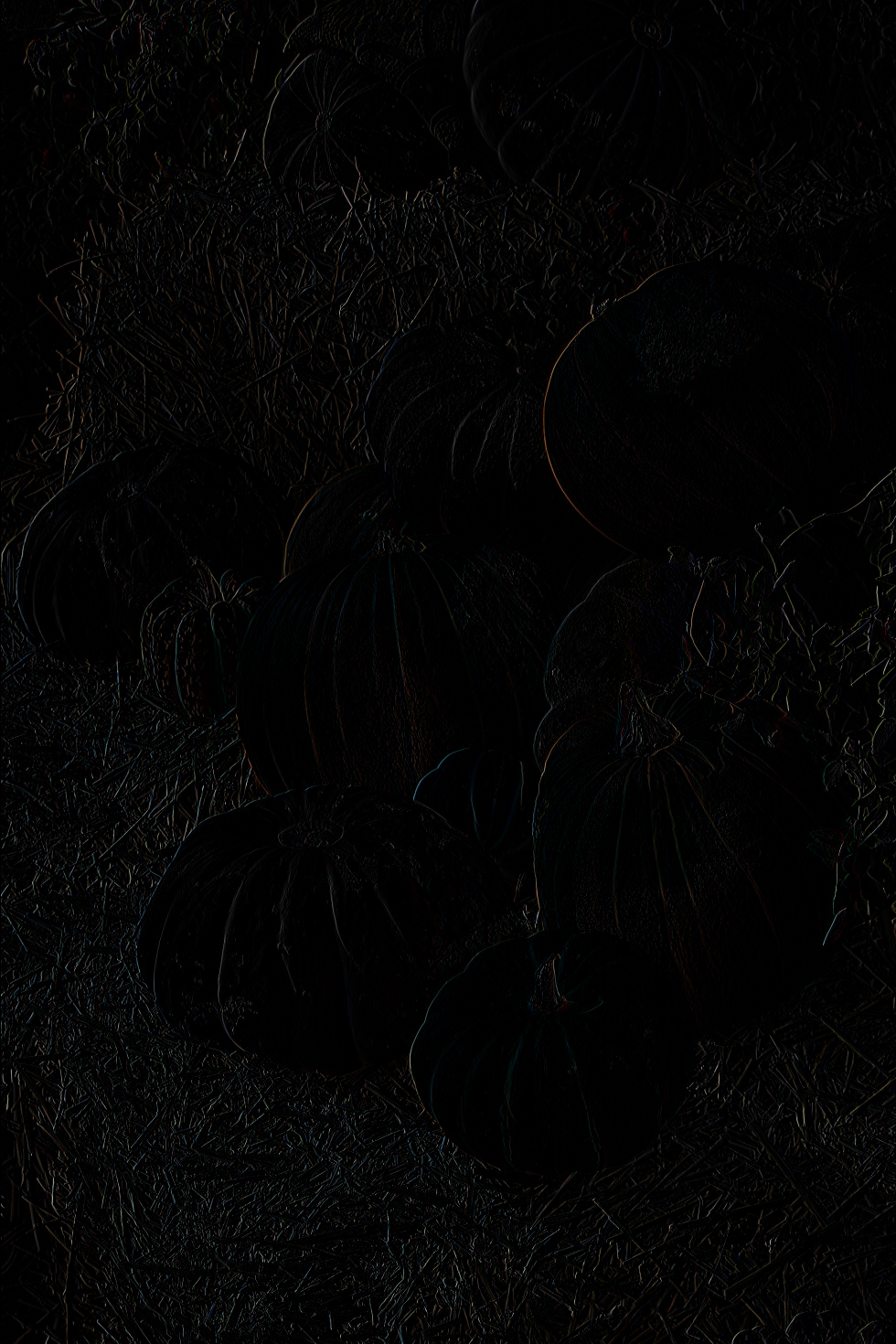Gaussian Blur:

``````
public static float[][] gaussianBlur = {
{1,4,6,4,1},
{4,16,24,16,4},
{6,24,36,24,6},
{4,16,24,16,4,},
{1,4,6,4,1}
};
``````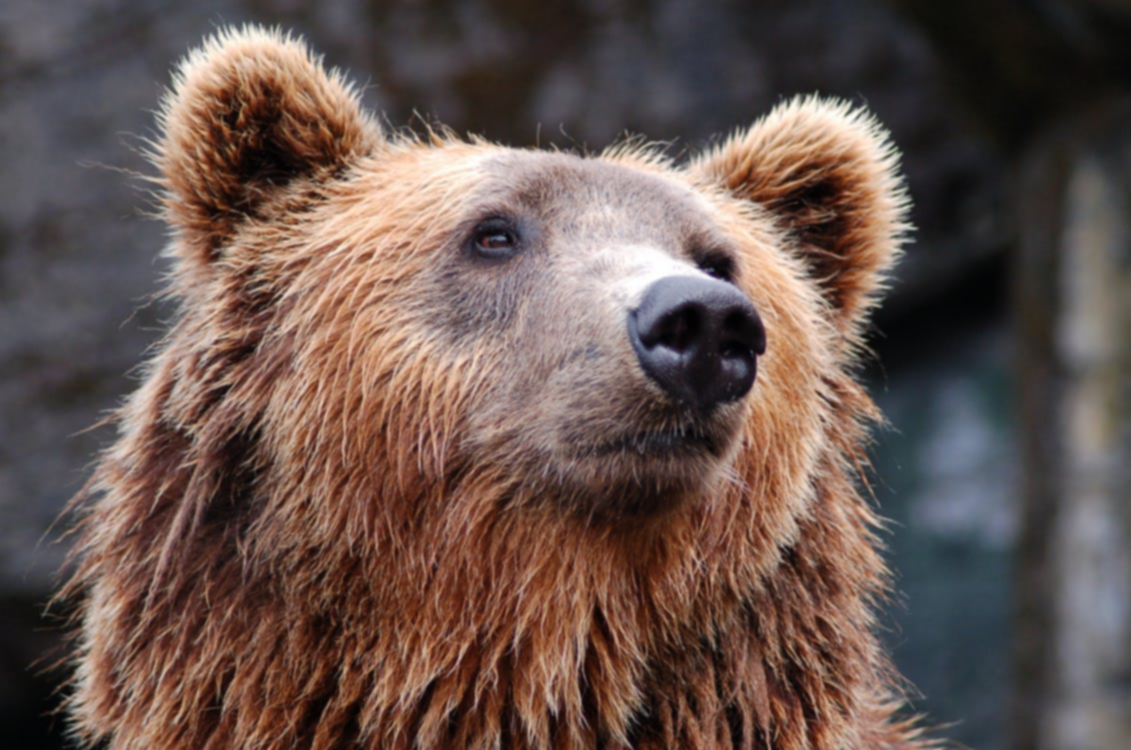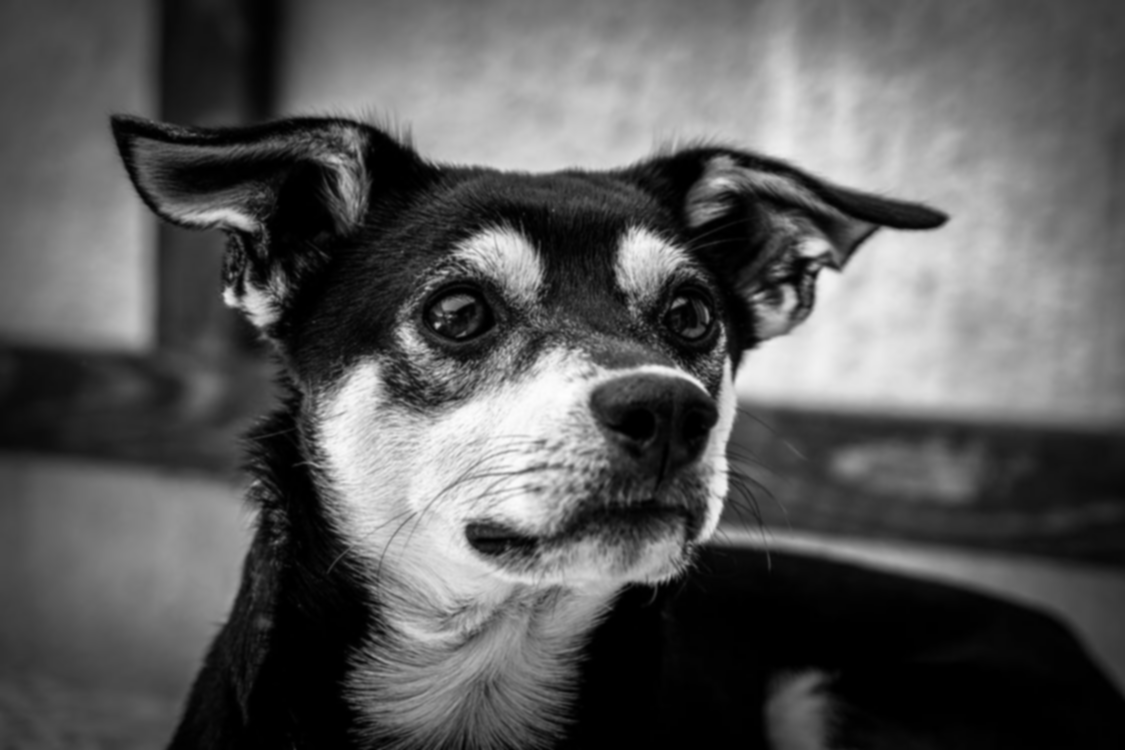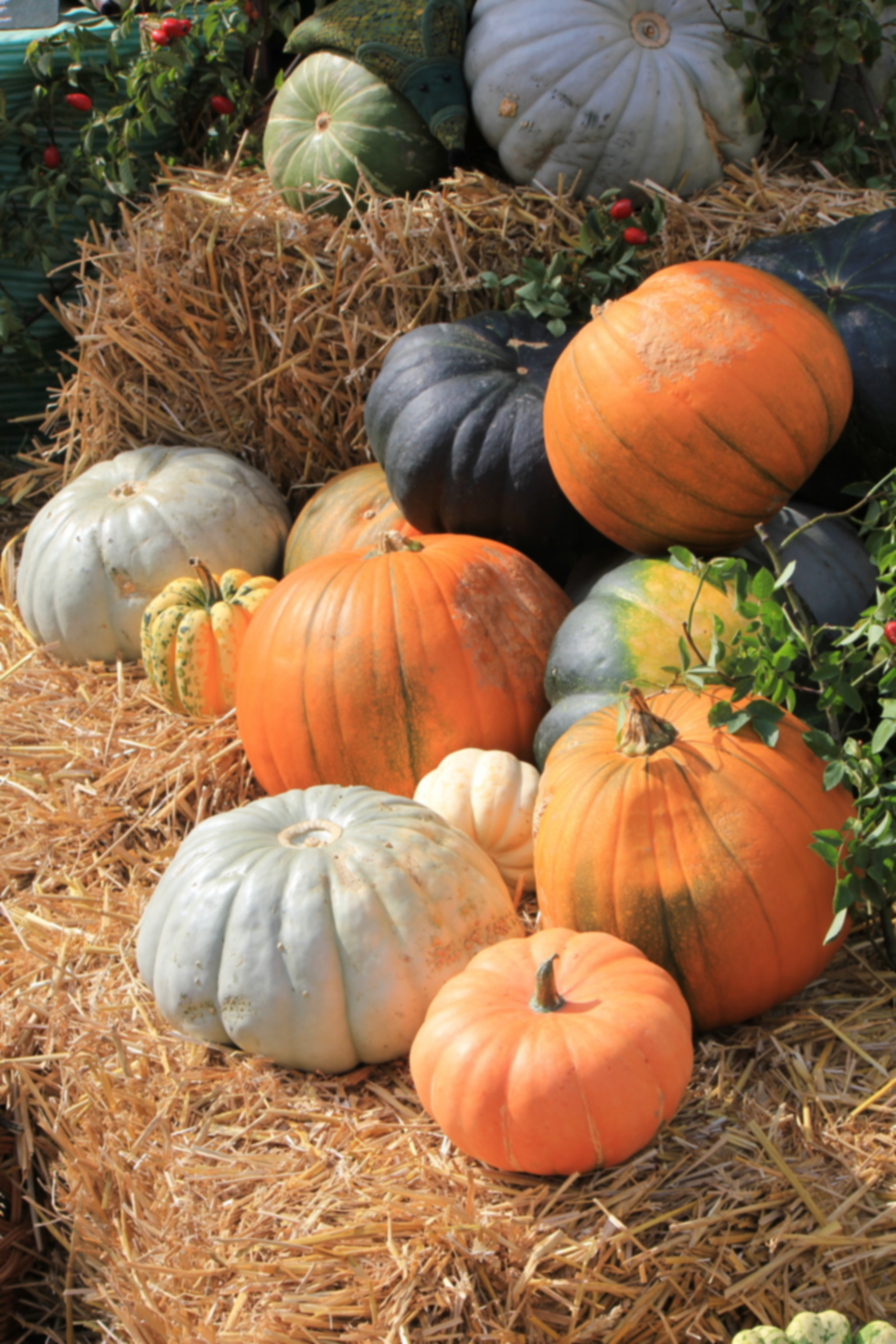Sharpen:

``````
public static float[][]sharpenGimp = {
{0,0,0,0,0},
{0,0,-1,0,0},
{0,-1,5,-1,0},
{0,0,-1,0,0},
{0,0,0,0,0,}
};
``````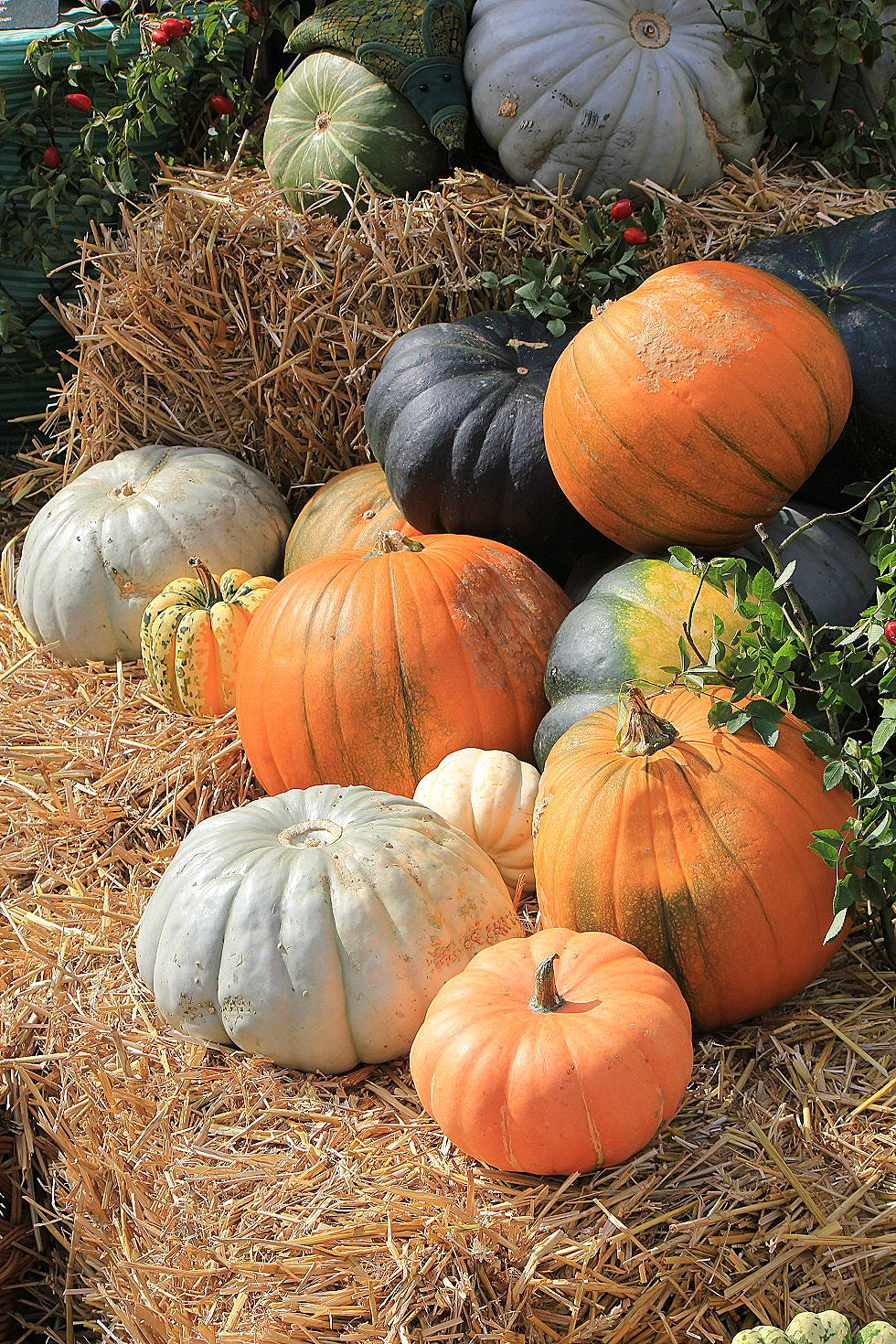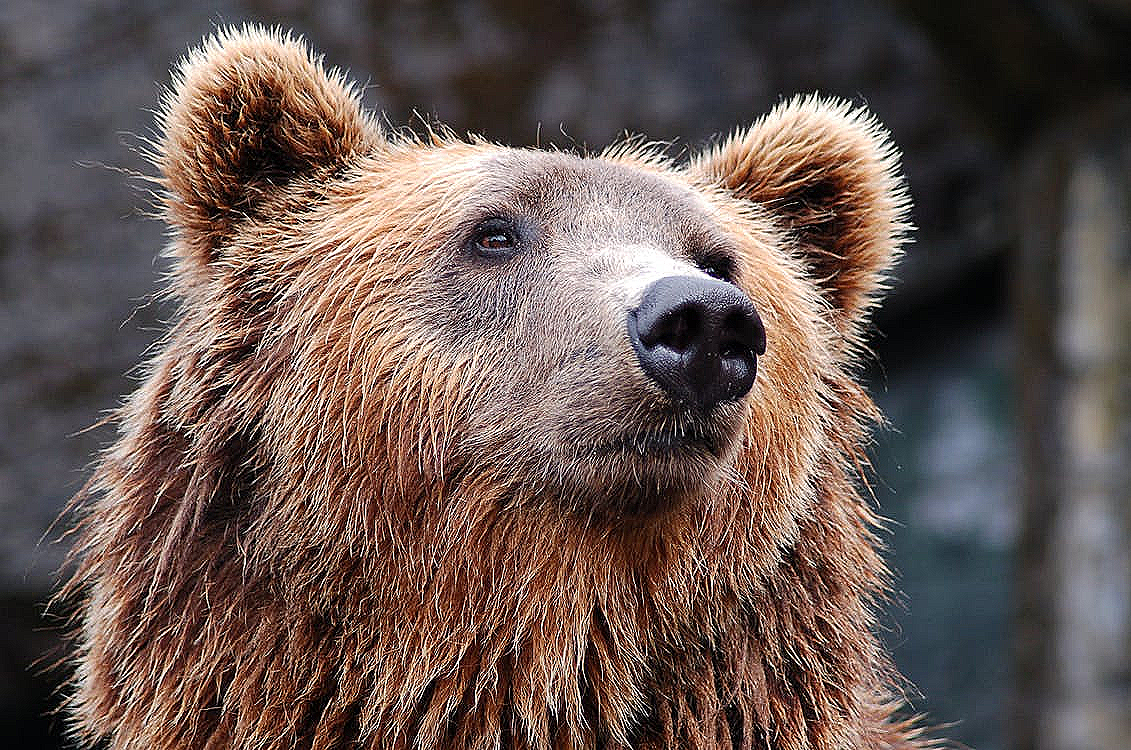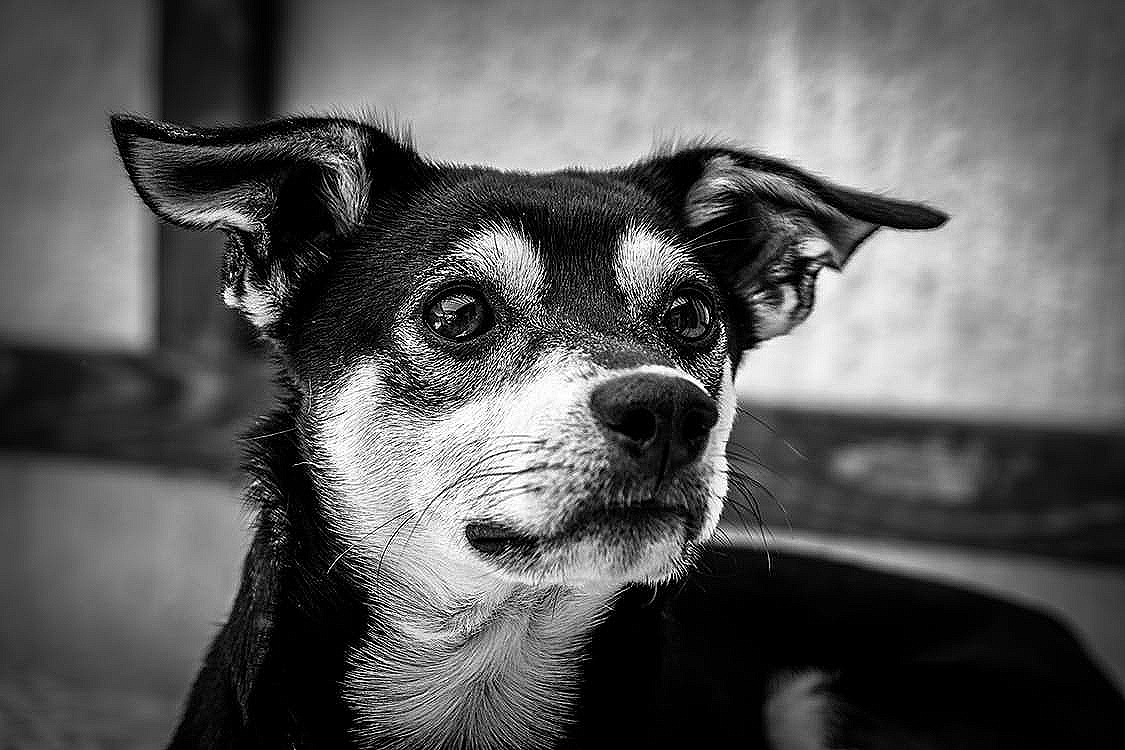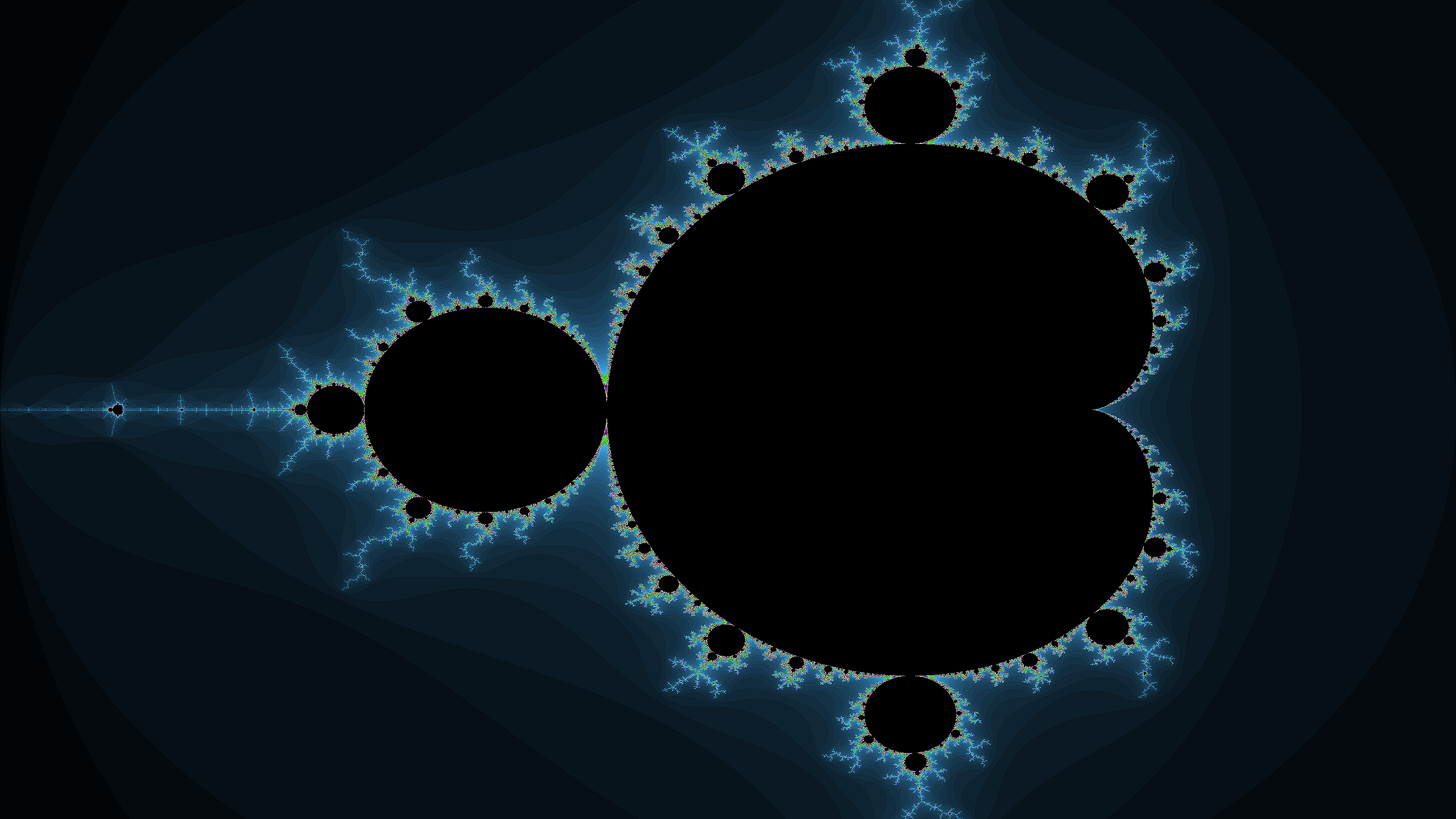- https://en.wikipedia.org/wiki/Kernel_(image_processing)
- an amazing visual explanation of kernel I found: http://setosa.io/ev/image-kernels/
- Gimp: https://docs.gimp.org/en/plug-in-convmatrix.html